# Measurements of inclusive jet spectra in pp and central Pb-Pb collisions at $\sqrt{s_{\rm{NN}}}$ = 5.02 TeV

This article reports measurements of the $p_{\rm{T}}$-differential inclusive jet cross-section in pp collisions at $\sqrt{s}$ = 5.02 TeV and the $p_{\rm{T}}$-differential inclusive jet yield in Pb-Pb 0-10% central collisions at $\sqrt{s_{\rm{NN}}}$ = 5.02 TeV. Jets were reconstructed at mid-rapidity with the ALICE tracking detectors and electromagnetic calorimeter using the anti-$k_{\rm{T}}$ algorithm. For pp collisions, we report jet cross-sections for jet resolution parameters $R=0.1-0.6$ over the range $20<~p_{\rm{T,jet}}<~140$ GeV/$c$, as well as the jet cross-section ratios of different $R$, and comparisons to two next-to-leading-order (NLO)-based theoretical predictions. For Pb-Pb collisions, we report the $R=0.2$ and $R=0.4$ jet spectra for $40<~p_{\rm{T,jet}}<~140$ GeV/$c$ and $60<~p_{\rm{T,jet}}<~140$ GeV/$c$, respectively. The scaled ratio of jet yields observed in Pb-Pb to pp collisions, $R_{\rm{AA}}$, is constructed, and exhibits strong jet quenching and a clear $p_{\rm{T}}$-dependence for $R=0.2$. No significant $R$-dependence of the jet $R_{\rm{AA}}$ is observed within the uncertainties of the measurement. These results are compared to several theoretical predictions.

Submitted to: PRC
e-Print: arXiv:1909.09718 | PDF | inSPIRE
CERN-EP-2019-200

Figures

## Figure 1

 Left: Unfolded \pp{} full jet cross-section at $\sqrt{s}=5.02$ TeV for $R=0.1-0.6$. No leading track requirement is imposed Right: Ratio of NLO+NLL+NP and POWHEG+PYTHIA8 tune A14 predictions to the measured data. The systematic uncertainties in the ratio are denoted by boxes, and are the quadratic sum of the systematic uncertainties in data and the predictions. Note that no systematic uncertainties for the non-perturbative correction in the NLO+NLL+NP prediction were included.## Figure 2

 Non-perturbative correction factor applied to parton-level NLO+NLL predictions, obtained from PYTHIA 8 tune A14 as the ratio of the inclusive jet spectrumat hadron-level with MPI compared to parton-level without MPI.## Figure 3

 Unfolded \pp{} jet cross-section ratios for various $R$. Top panels: ratios of $R=0.2$ to other radii. Bottom panels: ratios of $R=0.1$ to other radii The left panels include comparisons to POWHEG + PYTHIA8, and the right panels include comparisons to NLO+NLL+NP The experimental correlated systematic uncertainties and shape systematic uncertainties were combined in quadrature into a single systematic uncertainty Note that no systematic uncertainties for the non-perturbative correction in the NLO+NLL+NP prediction were included.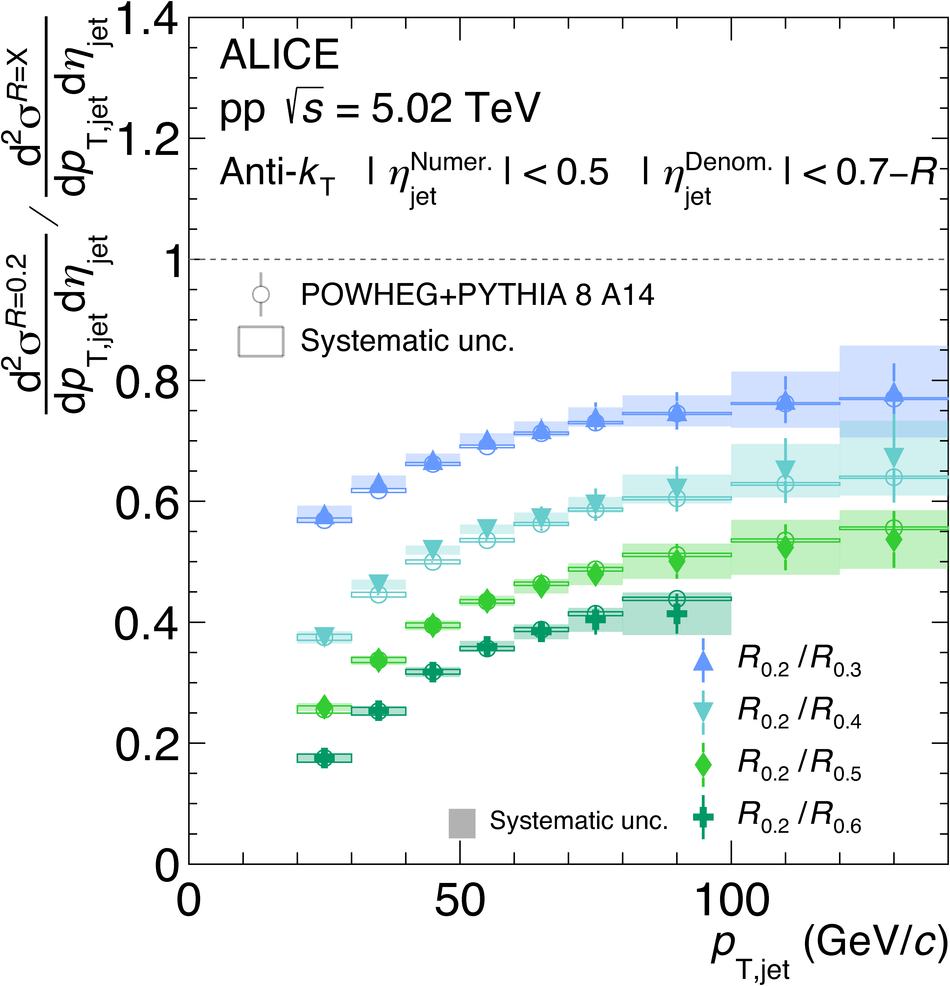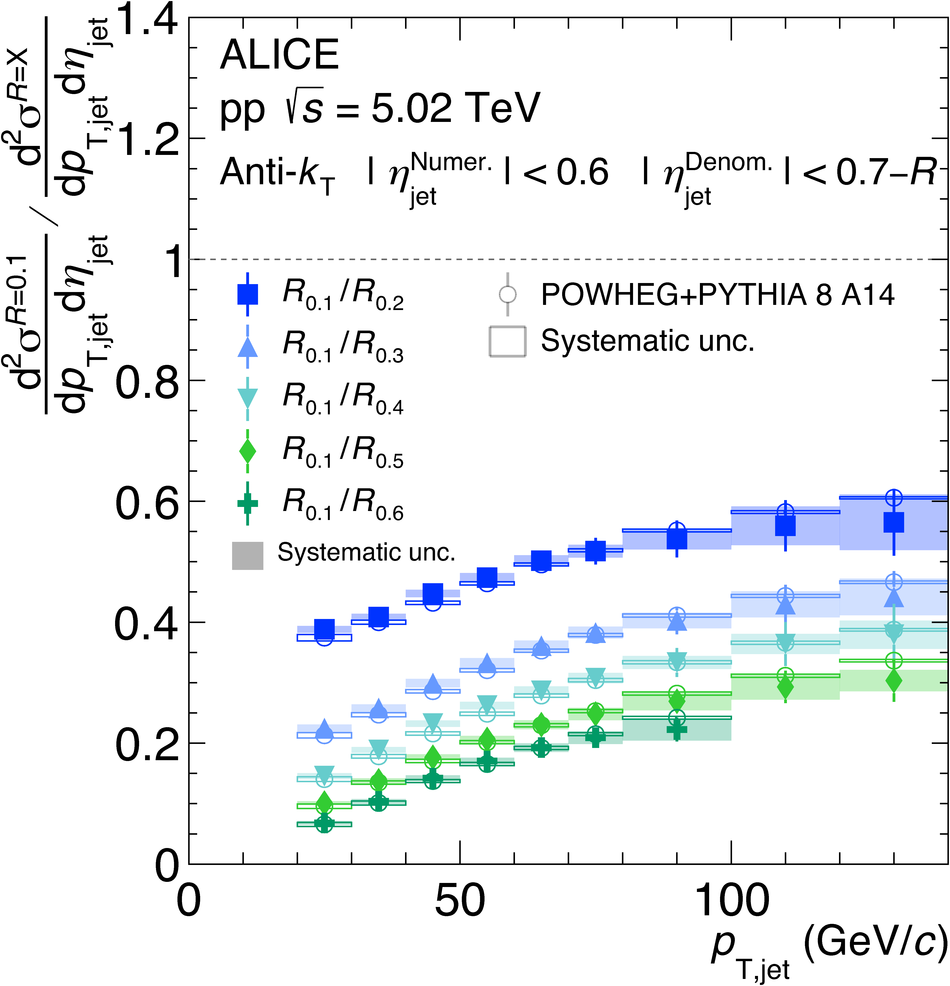## Figure 4

 Unfolded \pp{} and \PbPb{} full jet spectra at $\sqrts=5.02$ TeV for $R=0.2$ (left), with 5 \GeVc{} leading track requirement, and $R=0.4$ (right), with 7 \GeVc{} leading track requirement The \pp{} data points correspond to $\frac{\mathrm{d}^{2}\sigma}{\mathrm{d}\pTjet \mathrm{d}\etajet}$.## Figure 5

 Left: Ratio of the \pp{} jet cross-section with various leading charged particle requirements Right: Ratio of the $R=0.2$ \PbPb{} jet cross-section with a 7 \GeVc{} leading charged particle requirement compared to a 5 \GeVc{} leading charged particle requirement.## Figure 6

 Jet \Raa{} at $\sqrts=5.02$ TeV for $R=0.2$ (left) and $R=0.4$ (right). In the top panel a leading track requirement is imposed on the \pp{} reference, while in the bottom panel no such requirement is imposed on the \pp{} reference The combined \Taa{} uncertainty and \pp{} luminosity uncertainty of 2.8\% is illustrated as a band on the dashed line at $\Raa = 1$.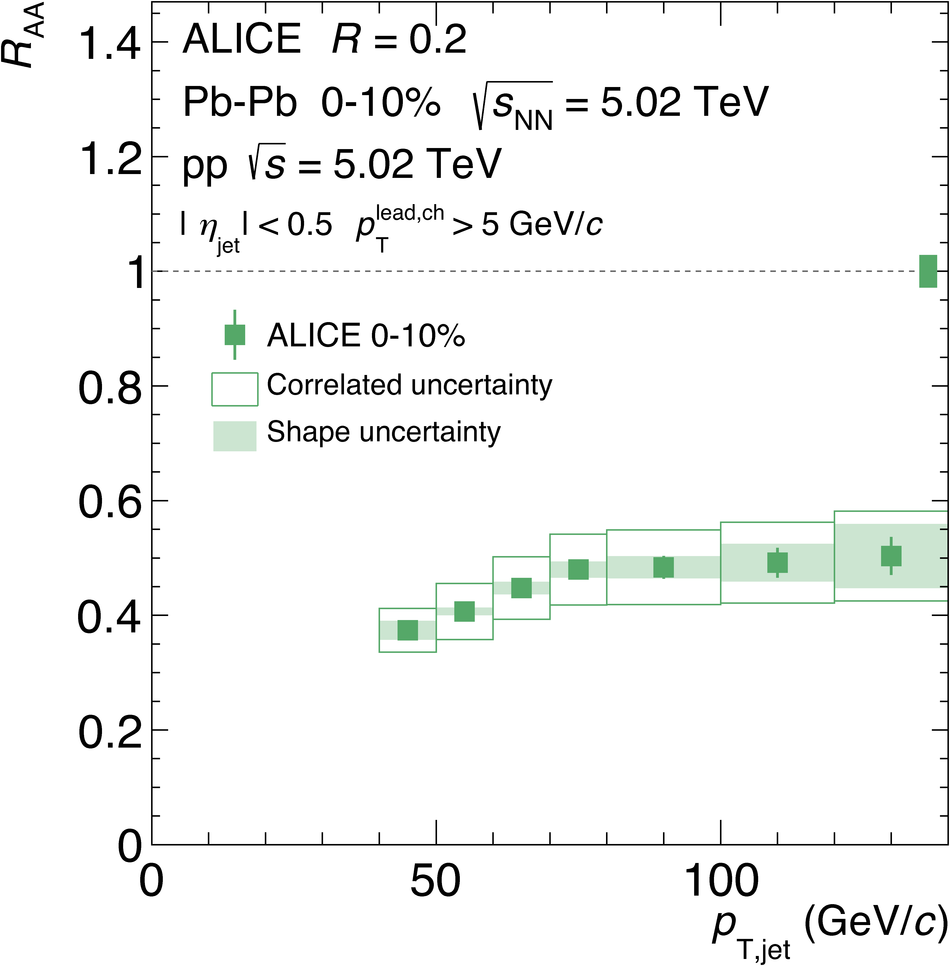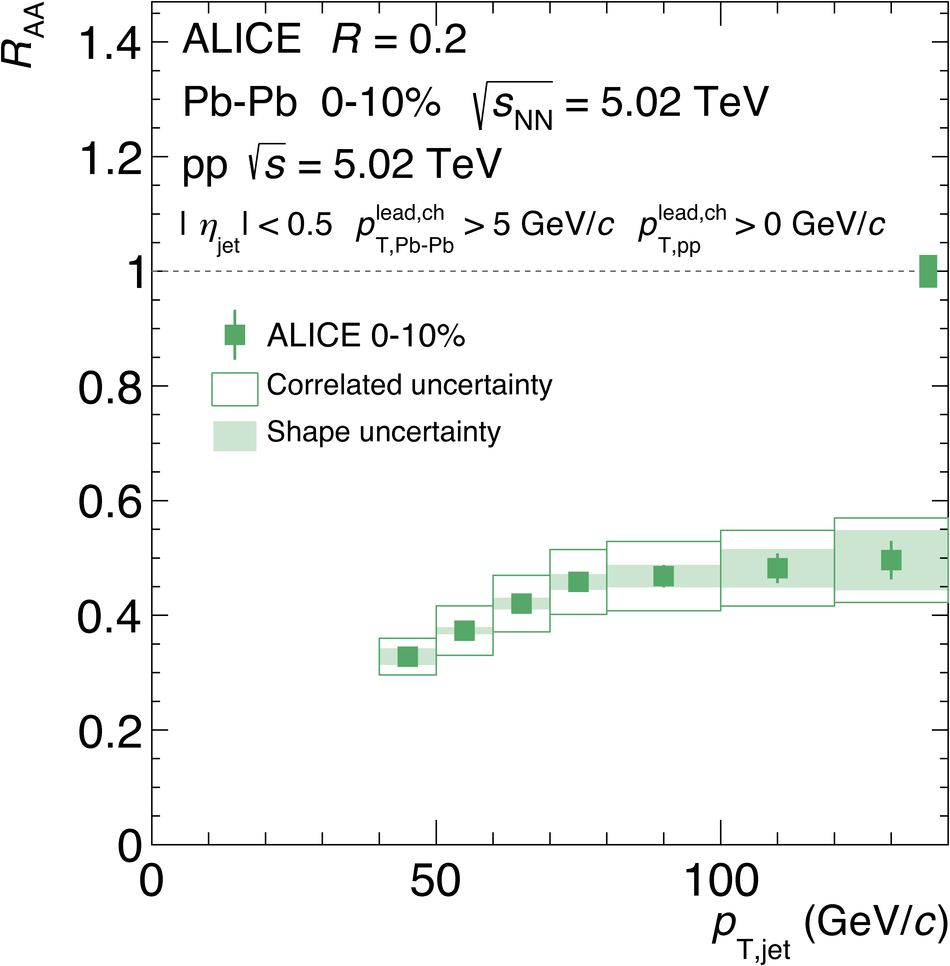## Figure 7

 Jet \Raa{} at $\sqrts=5.02$ TeV for $R=0.2$ (left) and $R=0.4$ (right) compared to LBT, SCET$_{G}$, Hybrid model, and JEWEL predictions. The combined \Taa{} uncertainty and \pp{} luminosity uncertainty of 2.8\% is illustrated as a band on the dashed line at $\Raa = 1$ Systematic uncertainties are only included for the SCET$_{G}$ and Hybrid model predictions; see text for details.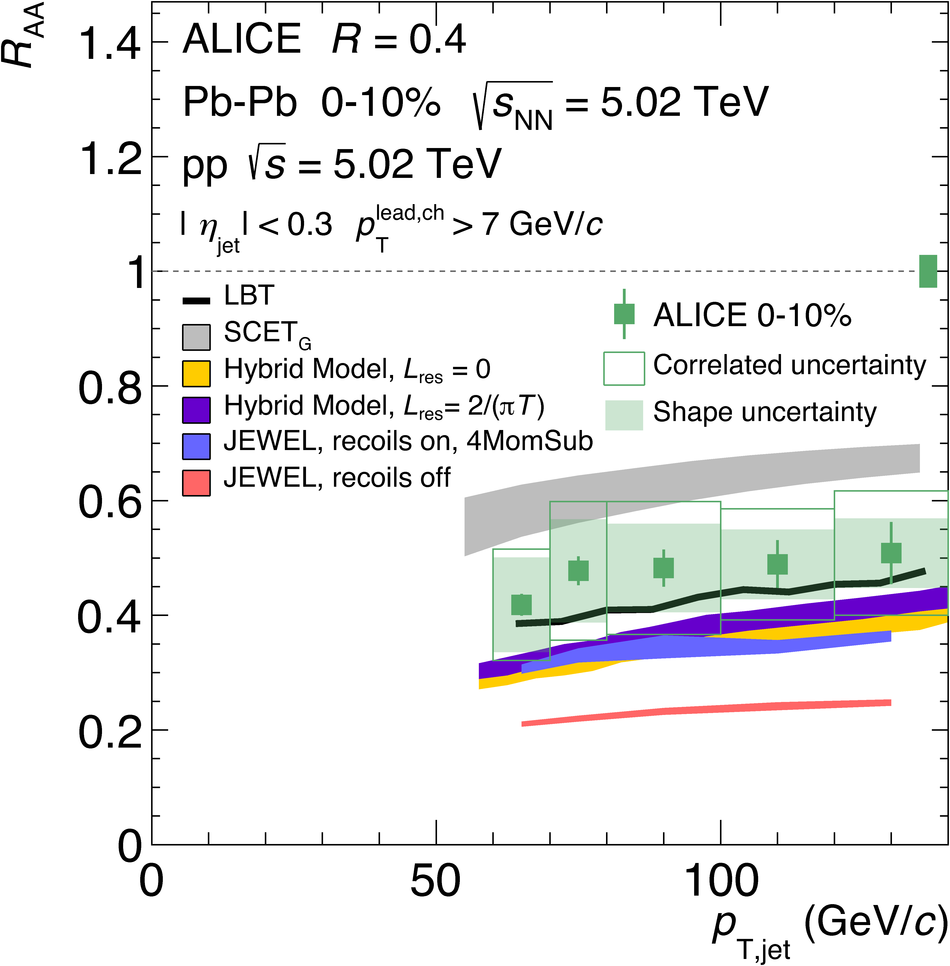## Figure 8

 Jet \Raa{} in 0-10\% central \PbPb{} collisions for $R=0.2$ (left) and $R=0.4$ (right) for all currently published experimental results. Closed markers denote $\sqrts=5.02$ TeV, and open markers denote $\sqrts=2.76$ TeV.